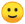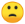# DFS vs BFS

Tuesday 09 of June, 2020.
By Miguel López MamaniAs a software engineer, there are moments in the analysis of a solution when it is important to know specific algorithms, such as tree-traversing algorithms. The objective of this article is to review two of the main search algorithms for connected graphs: depth-first search (DFS) and breadth-first search (BFS), both of which can be used to improve the efficiency of a program.

## Depth-First Search

A depth-first search (DFS) is a search algorithm that traverses nodes in a graph. It functions by expanding every one of the nodes it locates in a recurrent manner (from the parent node to the child nodes). When there are no more nodes to traverse, it returns to the previous node and repeats the process with every one of the neighboring nodes. It’s important to note that if it finds the node it is looking for before traversing all nodes, the search ends.

Depth-first searches are used when determining if one solution among many meets certain requirements; an example of a depth-first search could be determining the route a knight piece in chess must take in order to traverse all 64 squares on the board.

The following is a disconnected graph with eight nodes. The orange arrows indicate the node path taken by the DFS algorithm.The following shows the implementation of a depth-first search in C++, where the algorithm traverses the graph’s nodes using an iterator that begins on the first node and proceeds until the last entered node. Two variables are used “visitedNode” and “checkedNode” to organize the depth-first path; for this reason, recursion is used to traverse the nodes.

``````
void DFS(grafo &g,int s){
visitedNode[s]=true;
checkNode(s);
list<int>::iterator it;

for(it=g.sides[s].begin(); it!=g.sides[s].end(); ++it){
if(!visitedNode[*it]){
parentNode[*it]=s;
DFS(g,*it);
}else if(!checkedNode[*it]){
checkSide(s,*it);
}
}
checkedNode[s]=true;
}
``````

Additionally, 2 functions are called (“checkNode” and “checkSide”) to observe the node path, just like it’s shown in the following image:In the above image, the first 2 digits (8,8) represent the number of nodes and sides, followed by 8 pairs of digits that represent the connections between the nodes. Finally, the path of the DFS algorithm is shown from node 1 to node 8.

## DFS Applied

Depth-first search algorithms have several applications, which include:

• Finding connected nodes within a graph
• Topological organization of a directed acyclic graph
• Finding bridges between graph nodes
• Solving puzzles with a single solution, such as labyrinths
• Finding strongly connected nodes

A directed acyclic graph (a set of nodes where each node has a single direction that is not itself) can be organized topologically using a DFS algorithm. This can be used to organize activities that have some kind of dependency on each other, with the goal of achieving the most efficient execution of a list of activities.

Graph bridges are 2 nodes that are connected in such a way that in order to reach their respective ends, it is necessary to traverse one of the two nodes. In other words, if you remove one of the nodes, you will not be able to access the other node because it has been completely disconnected. This can be used to prioritize activities represented by the nodes.

If you are looking to solve a puzzle or labyrinth, this can be done using DFS; the subsequent steps of the solution can be represented by the nodes, where each node is dependent on the previous node. In short, each step of the puzzle’s solution will depend on the previous step.

In some cases, it’s important to know how certain activities or components are interconnected in order to reduce the dependency between activities or components. The objective is to organize activities or a group of components in the best way possible so the system is easier to understand; this can also be done using a DFS algorithm.

A breadth-first search (BFS) is an algorithm that traverses graph nodes. It begins at the root node (one of the nodes in the graph is chosen as the root) and then explores all its neighboring nodes. In the following example, each of the adjacent neighboring nodes is explored respectively until the whole graph is traversed. It’s important to note that if the algorithm finds the node it is looking for before traversing all nodes, the search ends.

A breadth-first search is used for algorithms for which it is crucial to choose the best possible path at all times.

The following is a disconnected graph where the orange arrows indicate the node path taken by the BFS algorithm.The following shows the implementation of a breadth-first search in C++, where the algorithm traverses the graph’s nodes using an iterator that begins on the first node and proceeds until the last entered node; it traverses all neighboring nodes before moving on to the child nodes. The breadth-first path is organized using two variables: “visitedNode” and “checkedNode”. For this reason, a queue data structure is used to visit all nodes in order of arrival. In other words, the algorithm processes the nodes that arrived first in the queue.

``````
void BFS(graph &g,int nodeStart){
int node;
queue<int> nodeQueue;
list<int>::iterator it;

visitedNode[nodeStart]=true;
nodeQueue.push(nodeStart);
while(!nodeQueue.empty()){
node=nodeQueue.front(),nodeQueue.pop();
checkedNode[node]=true;
checkNode(node);
for(it=g.sides[node].begin(); it!=g.sides[node].end(); ++it){
if(!visitedNode[*it]){
nodeQueue.push(*it),visitedNode[*it]=true;
parentNode[*it] = node;
}
if(!checkedNode[*it]) {
checkSide(node,*it);
}
}
}
}
``````

Additionally, 2 functions are called (“checkNode” and “checkSide”) to observe the node path, just like it’s shown in the following image:It’s important to note that in the previous image, the first two digits (8,8) represent the number of nodes and sides, followed by 8 pairs of digits that represent connections between nodes. Finally, the path taken by the BFS algorithm from node 1 to node 8 is shown.

## BFS Applied

A breadth-first search algorithm has several applications, which include:

• Finding the shortest path between 2 nodes, measured by the number of connected nodes
• Proving if a graph is bipartite (it can be divided into two parts)
• Finding the minimum expansion tree in an unweighted graph
• Creating a web crawler
• GPS navigation systems to find neighboring locations

In unweighted graphs (where nodes have no cost or weight), it is possible to use BFS algorithms to find the shortest path. If you have a set of activities represented by nodes and you want to reach a specific activity by executing the least possible number of activities, you can use this algorithm; in the case of a weighted graph, using the previous example where activities have a determined time frame, you can modify the BFS algorithm and obtain the Dijkstra algorithm (also known as Shortest Path First algorithm).

According to code theory in order to decode word-codes, it is possible to use BFS to test if the node graph is bipartite. This result, in turn, will verify if the information is corrupted or not. An example is a Tanner graph, where all the nodes on one side are represented by word-code digits and the nodes on the other side represent the combination of digits that are expected to add up to zero in a word-code that is free of errors.

Finding the minimum expansion tree in an unweighted graph is another way to use a BFS algorithm. If you want to traverse a series of places without going through the same place twice, you can use this algorithm.

If you want to create a website search engine like Google, you can access the DOM (Document Object Model) using a web crawler, an algorithm that usually applies breadth-first searches to traverse HTML tags; in this way, you can limit the level of searches in the DOM.

In GPS navigation systems, you can use BFS algorithms to find nearby places of interest. As an example, if you have a series of tourist attractions and services represented by nodes that are connected based on their geographical proximity, then you can create a list of nearby tourist attractions, which can be used to plan a trip or tour.

## Conclusion

The algorithms mentioned in this article can be used to find paths between two or more nodes depending on the nature of the problem. BFS algorithms can be used on Google maps to find optimal routes between tourist attractions or to search texts within a web page using a web crawler. DFS algorithms can be used to solve puzzles or games, such as labyrinths and chess.

If you are looking for a software partner who will work towards your business goals and success, then Avantica is your solution. We offer dedicated teams, team augmentation, and individual projects to our clients. We are constantly looking for the best methodologies in order to give you the best results.